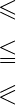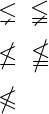# How to denote less than equal and not equal to symbol in LaTeX like ≤?

After the less than symbol, the most usable symbol is less than equal. Which is denoted by the ≤ symbol and for this latex has the default \leq command.

Symbol Less than or equal to symbol
Unicode/type U+2264 / Inequality
Package Default
Command \leq
Example a \leq ba  ≤  b
\documentclass{article}
\begin{document}
% You cannot directly use this symbol in text mode
$a \leq b$
$p \leq q$
\end{document}

Output :

a ≤ b
p ≤ q


In latex, in addition to the less than equal symbol, there are multiple symbols to represent the less than and equal symbols together. However, you need to use multiple packages and commands to identify each symbol. For example

\documentclass{article}
\usepackage{amssymb,mathabx}
\begin{document}
$\leqslant$
$\leqq$
$\eqslantless$
\end{document}

Output :## Less than not equal or not less than equal symbol in LaTeX.

Less than not equal and not less than equal represent two different symbols. So, look at the output below.

\documentclass{article}
\usepackage{amssymb}
\begin{document}
$\lneq \; \lneqq$
$\nleq \; \nleqq$
$\nleqslant$
\end{document}

Output :#### Md Jidan Mondal

LaTeX expert with over 10 years of experience in document preparation and typesetting. Specializes in creating professional documents, reports, and presentations using LaTeX.